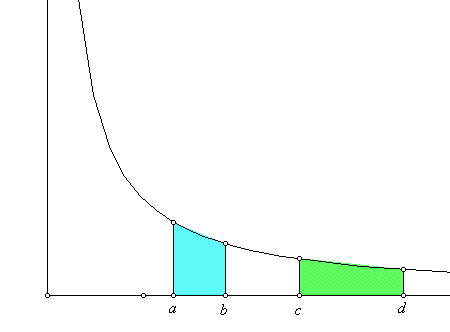Martin Flashman Department of Mathematics Humboldt State University The History of Logarithms: A glimpse of some highlights Thursday, February 4 SH 128 4:00 PM PreColloquium Tea and Refreshments Library 56 3:30 PM

Abstract:
Most students learn about logarithms in intermediate algebra and elementary functions using exponential functions and the concept of an inverse function.

The early history of logarithms had some less obvious (from todays viewpoint) origins related to geometric and arithmetic rates of change and finding areas related to hyperbolas.

Professor Flashman will examine some of this early history of logarithms including

(I) Napier's 1616 original definition and tables of logarithms,

(II) the work of Gregoire de Saint-Vincent in 1647, and

(III) Newton's 1676 approach to estimating some values of natural (or hyperbolic) logarithms.

# Part I Napier's logarithms  (1616, 1619)  (Text in html from the Netherlands).

A table (based on 100) that demonstrates the idea.

How would one use Napier's Tables:

Example: The rule of 3.
Suppose a/b = c/d.
Given any three of these values, find the fourth.

Napier's Theorem: If a/b=c/d then
NOG(a)- NOG(b) = NOG(c)-NOG(d)

Napier's logarithm tables.

Making Napier logarithm tables. (MS Excel)

How do Napier logarithms compare with modern logarithms?

# Part II. Gregoire de St. Vincent and hyperbolic areas.

In 1637, Descartes published  La Geometrie as an appendix to his Discours de la Methode.

By about 1640 the solution to the "area problem" for curves with equation  Y n = aX m  was known by Fermat  for all  integer cases except when n = 1, m = -1, i.e., Y = 1/X.

In 1647, Gregoire de St. Vincent showed:

If a/b=c/d
then
the area under the hyperbola above the interval [a,b] was equal to the area under the hyperbola above the interval [c,d].In 1649 Alfonso Antonio de Sarasa recognized this feature in Gregoire's work  and connected it to the properties of logarithms.

In particular he recognized the additive property of logarithms: that if areas are all measured using a = 1, then
the area determined by a product of two numbers , rs,  is equal to the sum of the areas determined by r and s separately.

# Part III . Newton's computations of hyperbolic logarithms.

In 1676 Newton wrote in a letter to Henry Oldenburg on some of his applications of series to estimating areas, in particular in estimating areas for the hyperbolic logarithm. This work was later clarified in Of the Method of Fluxions and Infinite Series which was published posthumously in 1737, ten years after Newton's death.

Newton's calculations.
A visualization of Newton's calculations using Geometer's Sketchpad.

The End